# Chapter 3 Graphics

In this chapter, we will introduce low-level functions that add graphics to the circle. Usages of most of these functions are similar as normal graphic functions (e.g. points(), lines()). Combination use of these functions can generate very complex circular plots.

All low-level functions accept sector.index and track.index arguments which indicate which cell the graphics are added in. By default the graphics are added in the “current” sector and “current” track, so it is recommended to use them directly inside panel.fun function. However, they can also be used in other places with explicitly specifying sector and track index. Following code shows an example of using ciros.points().

circos.track(..., panel.fun = function(x, y) {
circos.points(x, y)
})
circos.points(x, y, sector.index, track.index)

In this chapter, we will also discuss how to customize links and how to highlight regions in the circle.

## 3.1 Setting colors

Color is a major aesthetic element to map to the data points. In circlize there are two functions that provides customization of colors.

colorRamp2() provides an exact way for mapping continuous values. users specify a vector of break values and a vector of colors, all the other colors are linearly interpolated between the correspoding break values. In following example, we generate a color mapping which is symmetric to zero.

col_fun = colorRamp2(c(-2, 0, 2), c("blue", "white", "red"))
col_fun(seq(-5, 1, by = 1)) # all the values smaller than -2 are all mapped to blue
##  "#0000FFFF" "#0000FFFF" "#0000FFFF" "#0000FFFF" "#B38BFFFF" "#FFFFFFFF"
##  "#FF9E81FF"

rand_color() implements the algorithem of randomColor.js. See the following example:

par(mar = c(1, 1, 1, 1))
plot(NULL, xlim = c(1, 10), ylim = c(1, 8), axes = FALSE, ann = FALSE)
points(1:10, rep(1, 10), pch = 16, cex = 5,
col = rand_color(10, luminosity = "random"))
points(1:10, rep(2, 10), pch = 16, cex = 5,
col = rand_color(10, luminosity = "bright"))
points(1:10, rep(3, 10), pch = 16, cex = 5,
col = rand_color(10, luminosity = "light"))
points(1:10, rep(4, 10), pch = 16, cex = 5,
col = rand_color(10, luminosity = "dark"))
points(1:10, rep(5, 10), pch = 16, cex = 5,
col = rand_color(10, hue = "red", luminosity = "bright"))
points(1:10, rep(6, 10), pch = 16, cex = 5,
col = rand_color(10, hue = "green", luminosity = "bright"))
points(1:10, rep(7, 10), pch = 16, cex = 5,
col = rand_color(10, hue = "blue", luminosity = "bright"))
points(1:10, rep(8, 10), pch = 16, cex = 5,
col = rand_color(10, hue = "monochrome", luminosity = "bright"))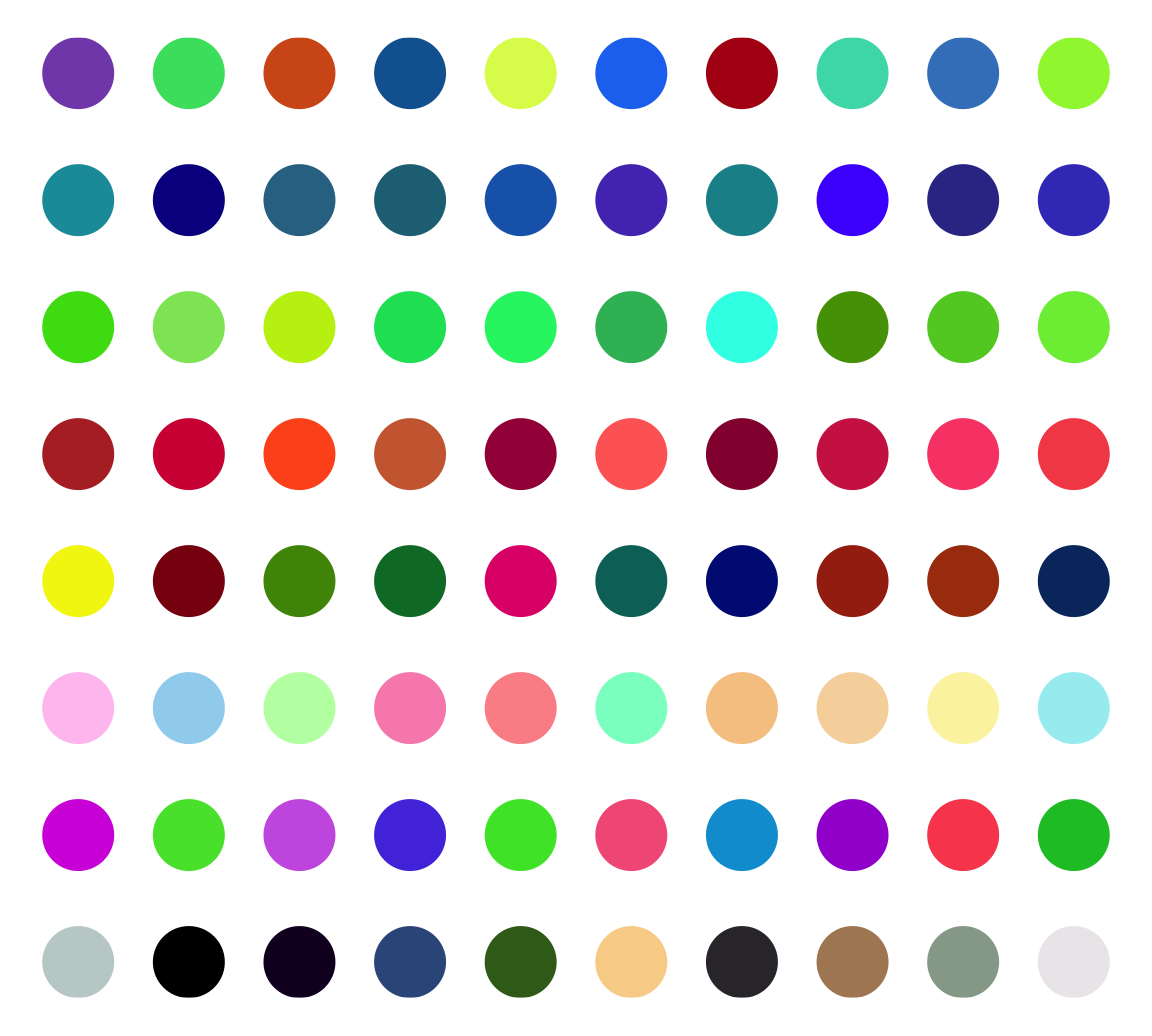Actually there is another function col2value() which can convert back to the values highly approximate to the original values from a vector of colors.

col_fun = colorRamp2(c(-2, 0, 2), c("blue", "white", "red"))
value = seq(-2, 2, by = 0.2)
value
##   -2.0 -1.8 -1.6 -1.4 -1.2 -1.0 -0.8 -0.6 -0.4 -0.2  0.0  0.2  0.4  0.6  0.8
##   1.0  1.2  1.4  1.6  1.8  2.0
col = col_fun(value)
col2value(col, col_fun = col_fun)
##   -2.000000e+00 -1.795620e+00 -1.595206e+00 -1.391406e+00 -1.189672e+00
##   -9.870220e-01 -7.853407e-01 -5.861849e-01 -3.901391e-01 -1.985154e-01
##  -7.401487e-17  1.761338e-01  3.592285e-01  5.454043e-01  7.429882e-01
##   9.423823e-01  1.150753e+00  1.359655e+00  1.575352e+00  1.785834e+00
##   2.000000e+00

## 3.2 Points

Adding points by circos.points() is similar as points() function. Possible usages are:

circos.points(x, y)
circos.points(x, y, sector.index, track.index)
circos.points(x, y, pch, col, cex)

There is a companion function circos.trackPoints() which adds points to all sectors in a same track simultaneously. The input of circos.trackPoints() must contain a vector of categorical factors, a vector of x values and a vector of y values. X values and y values are split by the categorical variable and corresponding subset of x and y values are internally sent to circos.points(). circos.trackPoints() adds points to the “current” track by default which is the most recently created track. Other tracks can also be selected by explictly setting track.index argument.

circos.track(...)
circos.trackPoints(sectors, x, y)

circos.trackPoints() is simply implemented by circos.points() with a for loop. However, it is more recommended to directly use circos.points() and panel.fun which provides great more flexibility. Actually following code is identical to above code.

circos.track(sectors, x, y, panel.fun = function(x, y) {
circos.points(x, y)
})

Other low-level functions also have their companion circos.track*() function. The usage is same as circos.trackPoints() and they will not be further discussed in following sections.

## 3.3 Lines

Adding lines by circos.lines() is similar as lines() function. One additional feature is that the areas under or above the lines can be filled by specifing area argument to TRUE. Position of the baseline can be set to a pre-defined string of bottom or top, or a numeric value which is the position on y-axis. When area is set to TRUE, col controls the filled color and border controls the color for the borders.

baseline argument is also workable when lty is set to "h". Note when lty is set to "h", graphic parameters such as col can be set as a vector with same length as x. Figure 3.1 illustrates supported lty settings and area/baseline settings.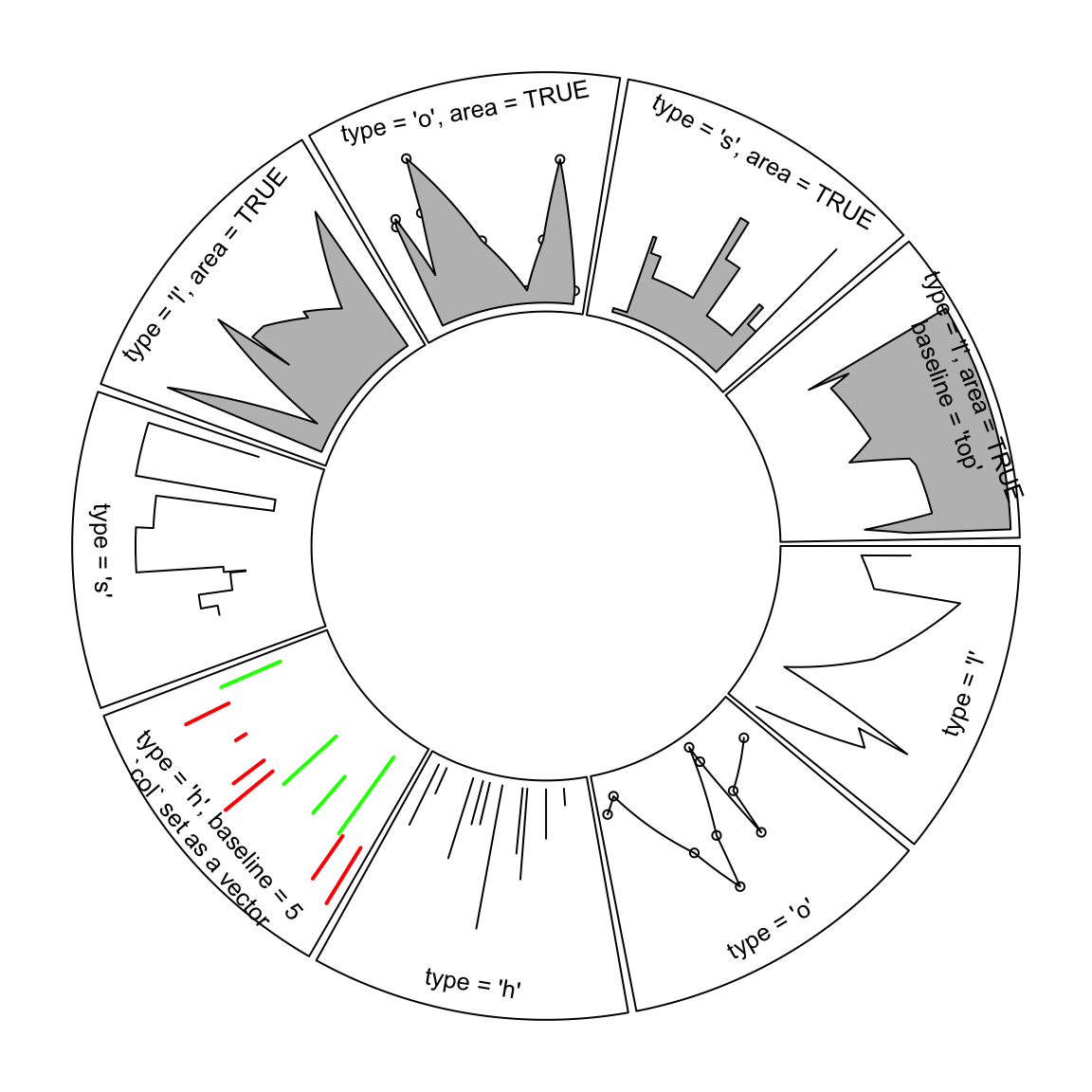Figure 3.1: Line styles and areas supported in circos.lines()

Straight lines are transformed to curves when mapping to the circular layout (Figure 3.2). Normally, curves are approximated by a series of segments of straight lines. With more and shorter segments, there is better approximation, but with larger size if the figures are generated into e.g. PDF files, especially for huge dataset. Default length of segments in circlize is a balance between the quality and size of the figure. You can set the length of the unit segment by unit.circle.segments option in circos.par(). The length of the segment is calculated as the length of the unit circle (2$$\pi$$) divided by unit.circle.segments. In some scenarios, actually you don’t need to segment the lines such as radical lines, then you can set straight argument to TRUE to get rid of unnecessary segmentations.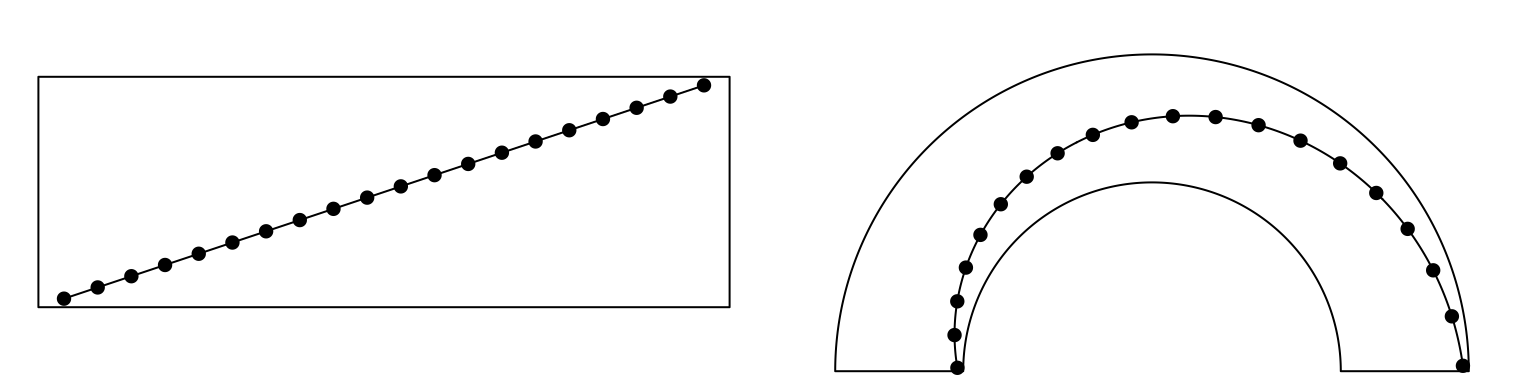Figure 3.2: Transformation of straight lines into curves in the circle.

Possible usages for circos.lines() are:

circos.lines(x, y)
circos.lines(x, y, sector.index, track.index)
circos.lines(x, y, col, lwd, lty, type, straight)
circos.lines(x, y, col, area, baseline, border)

## 3.4 Segments

Line segments can be added by circos.segments() function. The usage is similar as segments(). Radical segments can be added by setting straight to TRUE. An example is in Figure 3.3.

circos.segments(x0, y0, x1, y1)
circos.segments(x0, y0, x1, y1, straight)
circos.initialize(letters[1:8], xlim = c(0, 1))
circos.track(ylim = c(0, 1), track.height = 0.3, panel.fun = function(x, y) {
x = seq(0.2, 0.8, by = 0.2)
y = seq(0.2, 0.8, by = 0.2)

circos.segments(x, 0.1, x, 0.9)
circos.segments(0.1, y, 0.9, y)
})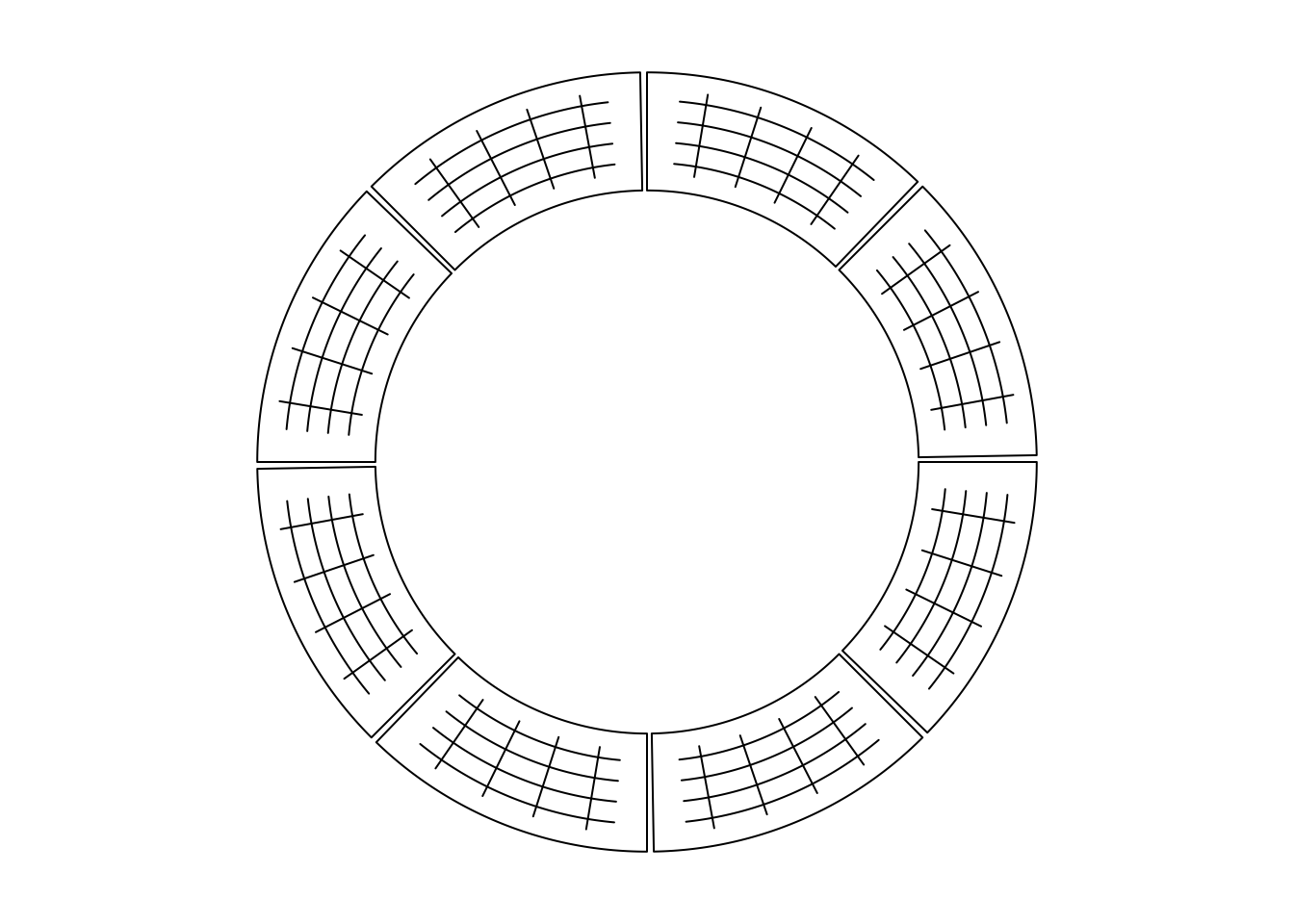Figure 3.3: Draw segments.

circos.clear()

## 3.5 Text

Adding text by circos.text() is similar as text() function. Text is added on the plot for human reading, thus, when putting the text on the circle, the facing of text is very important. circos.text() supports seven facing options which are inside, outside, clockwise, reverse.clockwise, downward, bending.inside and bending.outside. Please note for bending.inside and bending.outside, currently, single line text is only supported. If you want to put bended text into two lines, you need to split text into two lines and add each line by circos.text() separately. The different facings are illustrated in Figure 3.4.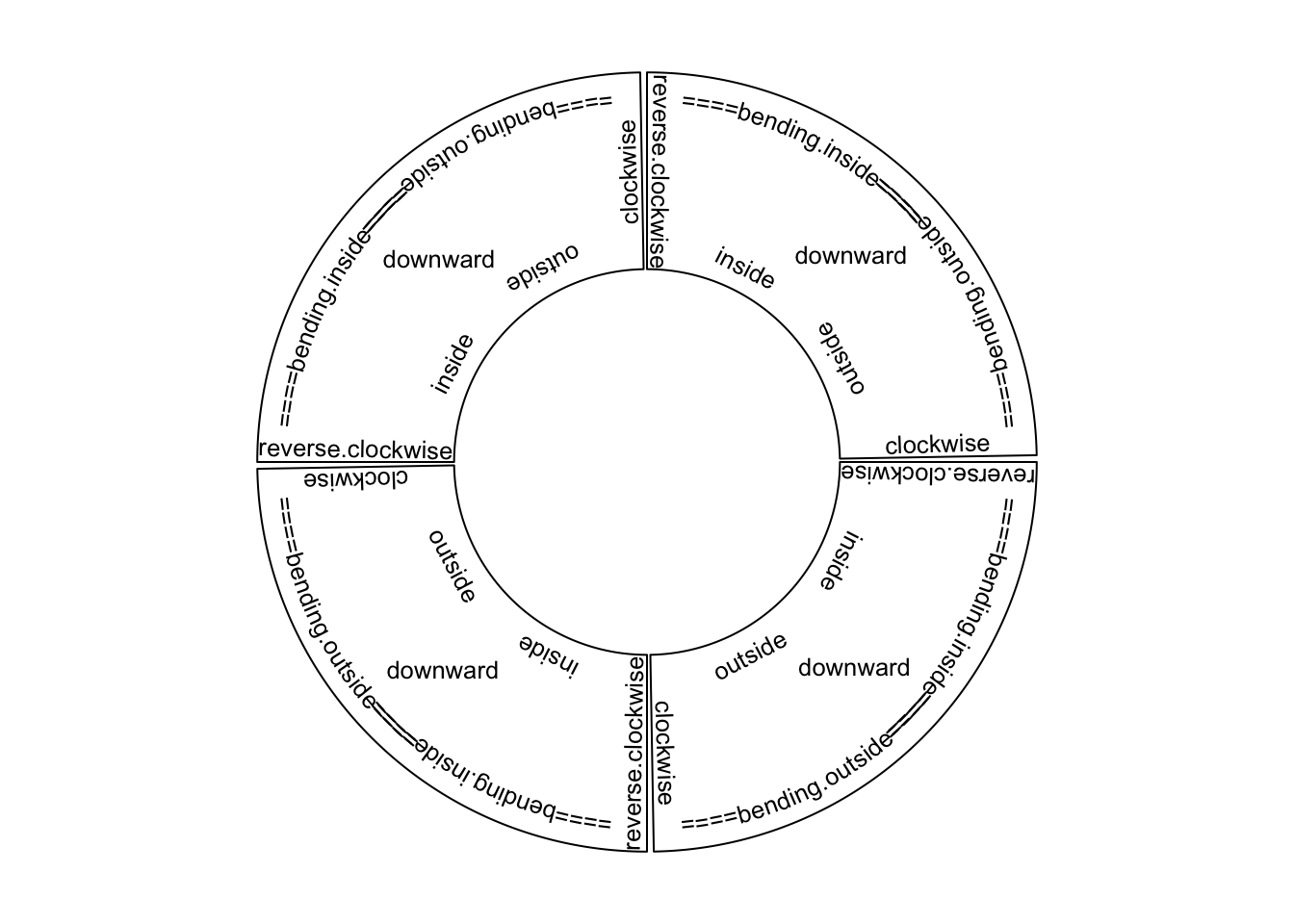Figure 3.4: Text facings.

Possible usages for circos.text() are:

circos.text(x, y, labels)
circos.text(x, y, labels, sector.index, track.index)
circos.text(x, y, labels, facing, niceFacing, adj, cex, col, font)

If, e.g., facing is set to inside, text which is on the bottom half of the circle is still facing to the top and hard to read. To make text more easy to read and not to hurt readers’ neck too much, circos.text() provides niceFacing option which automatically adjust text facing according to their positions in the circle. niceFacing only works for facing value of inside, outside, clockwise, reverse.clockwise, bending.inside and bending.outside.

When niceFacing is on, adj is also adjusted according to the corresponding facings. Figure 3.5 illustrates text positions under different settings of adj and facing. The red dots are the positions of the texts.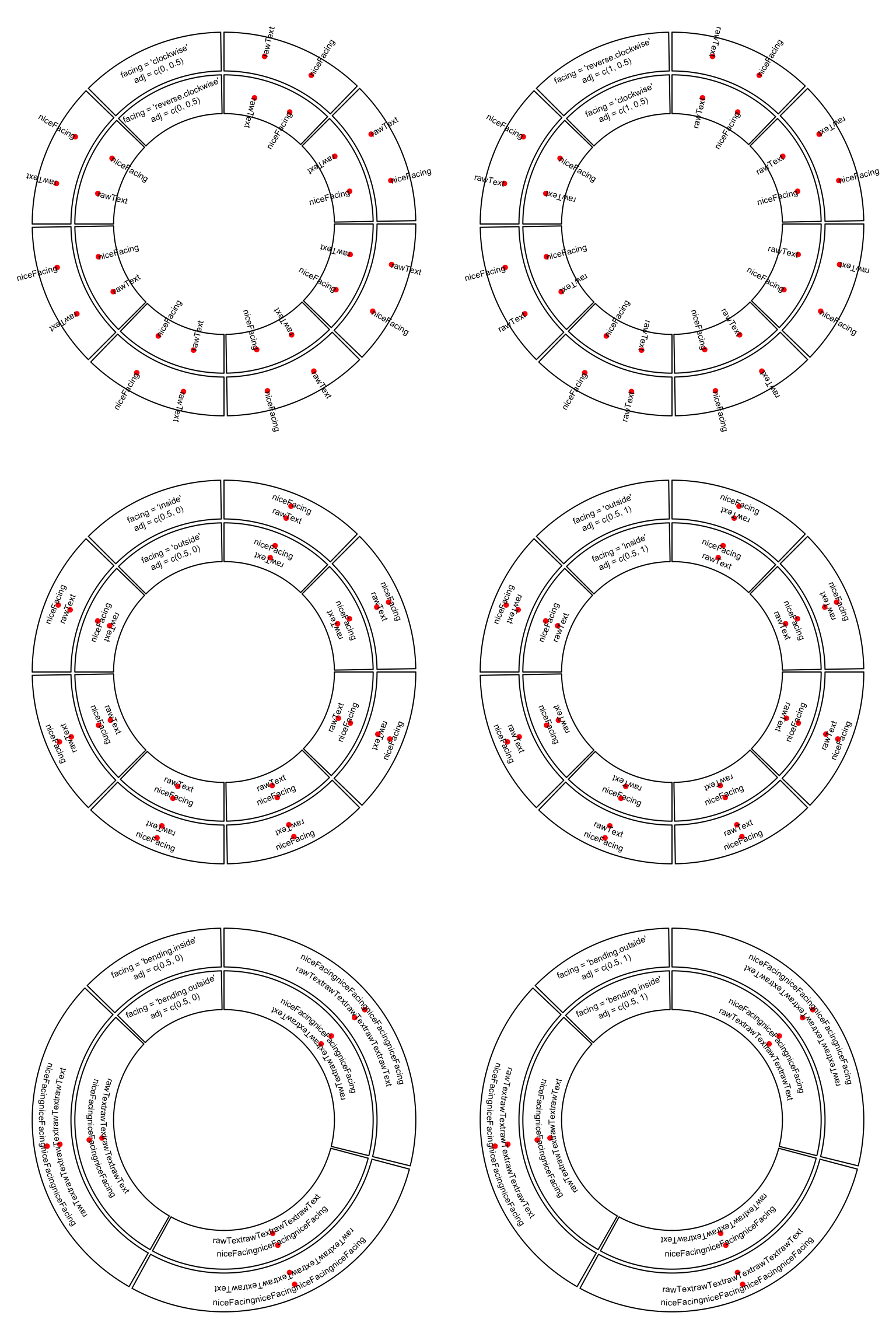Figure 3.5: Human easy text facing.

adj is internally passed to text(), thus, it actually adjusts text positions either horizontally or vertically (in the canvas coordinate). If the direction of the offset is circular, the offset value can be set as degrees that the position of the text is adjusted by wrapping the offset by degree().

circos.text(x, y, labels, adj = c(0, degree(5)), facing = "clockwise")

As circos.text() is applied in the data coordiante, offset can be directly added to x or/and y as a value measured in the data coordinate. An absolute offset can be set by using e.g. mm_x() (in x direction) and mm_y() (in y direction).

circos.text(x + mm_x(2), y + mm_y(2), labels)

## 3.6 Rectangles and polygons

Theoretically, circular rectangles and polygons are all polygons. If you imagine the plotting region in a cell as Cartesian coordinate, then circos.rect() draws rectangles. In the circle, the up and bottom edge become two arcs. Note this function can be vectorized.

circos.rect(xleft, ybottom, xright, ytop)
circos.rect(xleft, ybottom, xright, ytop, sector.index, track.index)
circos.rect(xleft, ybottom, xright, ytop, col, border, lty, lwd)

circos.polygon() draws a polygon through a series of points in a cell. Please note the first data point must overlap to the last data point.

circos.polygon(x, y)
circos.polygon(x, y, col, border, lty, lwd)

In Figure 3.6, the area of standard deviation of the smoothed line is drawn by circos.polygon(). Source code can be found in the Examples section of the circos.polygon() help page.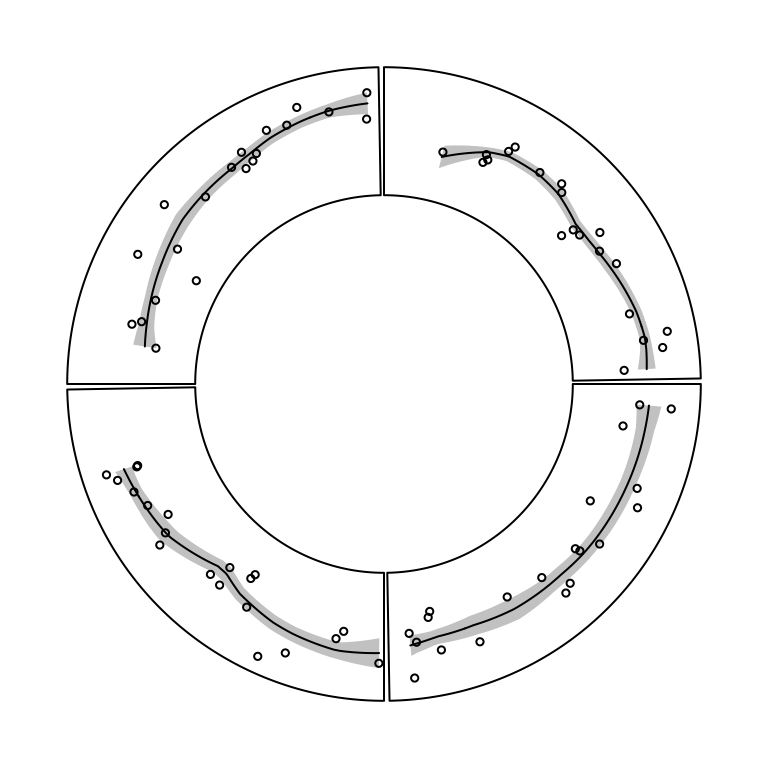Figure 3.6: Area of standard deviation of the smoothed line.

## 3.7 Axes

Mostly, we only draw x-axes on the circle. circos.axis() or circos.xaxis() privides options to customize x-axes which are on the circular direction. It supports basic functionalities as axis() such as defining the breaks and corresponding labels. Besides that, the function also supports to put x-axes to a specified position on y direction, to position the x-axes facing the center of the circle or outside of the circle, and to customize the axes ticks. The at and labels arguments can be set to a long vector that the parts which exceed the maximal value in the corresponding cell are removed automatically. The facing of labels text can be optimized by labels.niceFacing (by default it is TRUE).

Figure 3.7 illustrates different settings of x-axes. The explanations are as follows:

• a: Major ticks are calculated automatically, other settings are defaults.
• b: Ticks are pointing to inside of the circle, facing of tick labels is set to outside.
• c: Position of x-axis is bottom in the cell.
• d: Ticks are pointing to the inside of the circle, facing of tick labels is set to reverse.clockwise.
• e: manually set major ticks and also set the position of x-axis.
• f: replace numeric labels to characters, with no minor ticks.
• g: No ticks for both major and minor, facing of tick labels is set to reverse.clockwise.
• h: Number of minor ticks between two major ticks is set to 2. Length of ticks is longer. Facing of tick labels is set to clockwise.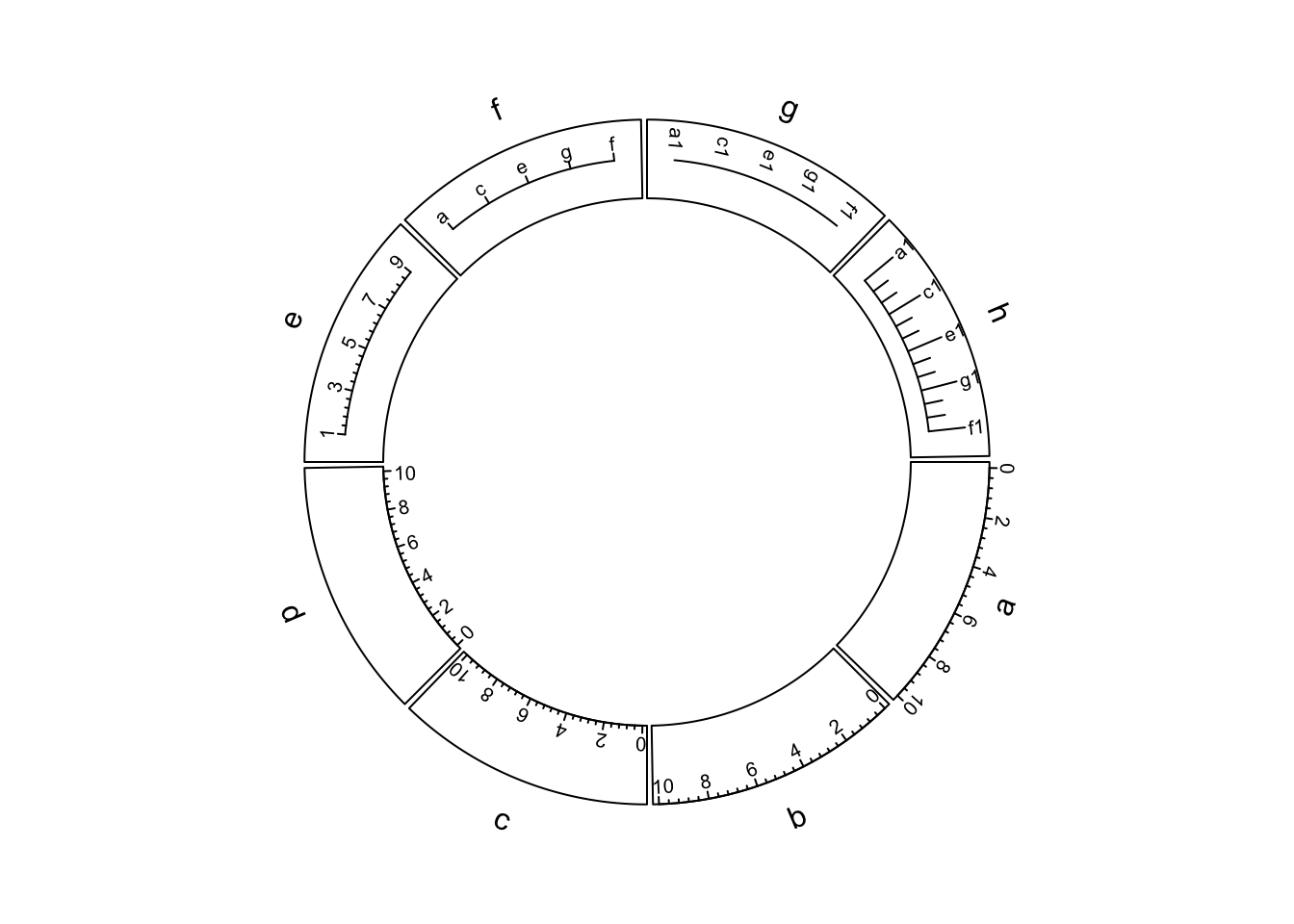Figure 3.7: X-axes

As you may notice in the above figure, when the first and last axis labels exceed data ranges on x-axis in the corresponding cell, their positions are automatically adjusted to be shifted inwards in the cell.

Possible usage of circos.axis() is as follows. Note h can be bottom, top or a numeric value, and major.tick.length can be set with mm_y()/cm_y()/inch_y().

circos.axis(h)
circos.axis(h, sector.index, track.index)
circos.axis(h, major.at, labels, major.tick, direction)
circos.axis(h, major.at, labels, major.tick, labels.font, labels.cex,
labels.facing, labels.niceFacing)
circos.axis(h, major.at, labels, major.tick, minor.ticks,
major.tick.length, lwd)

Y-axis is also supported by circos.yaxis(). The usage is similar as circos.axis() One thing that needs to be note is users need to manually adjust gap.degree/gap.after in circos.par() to make sure there are enough spaces for y-axes. (Figure 3.8)

circos.yaxis(side) # break values are automatically calculated
circos.yaxis(side, at, labels, sector.index, track.index)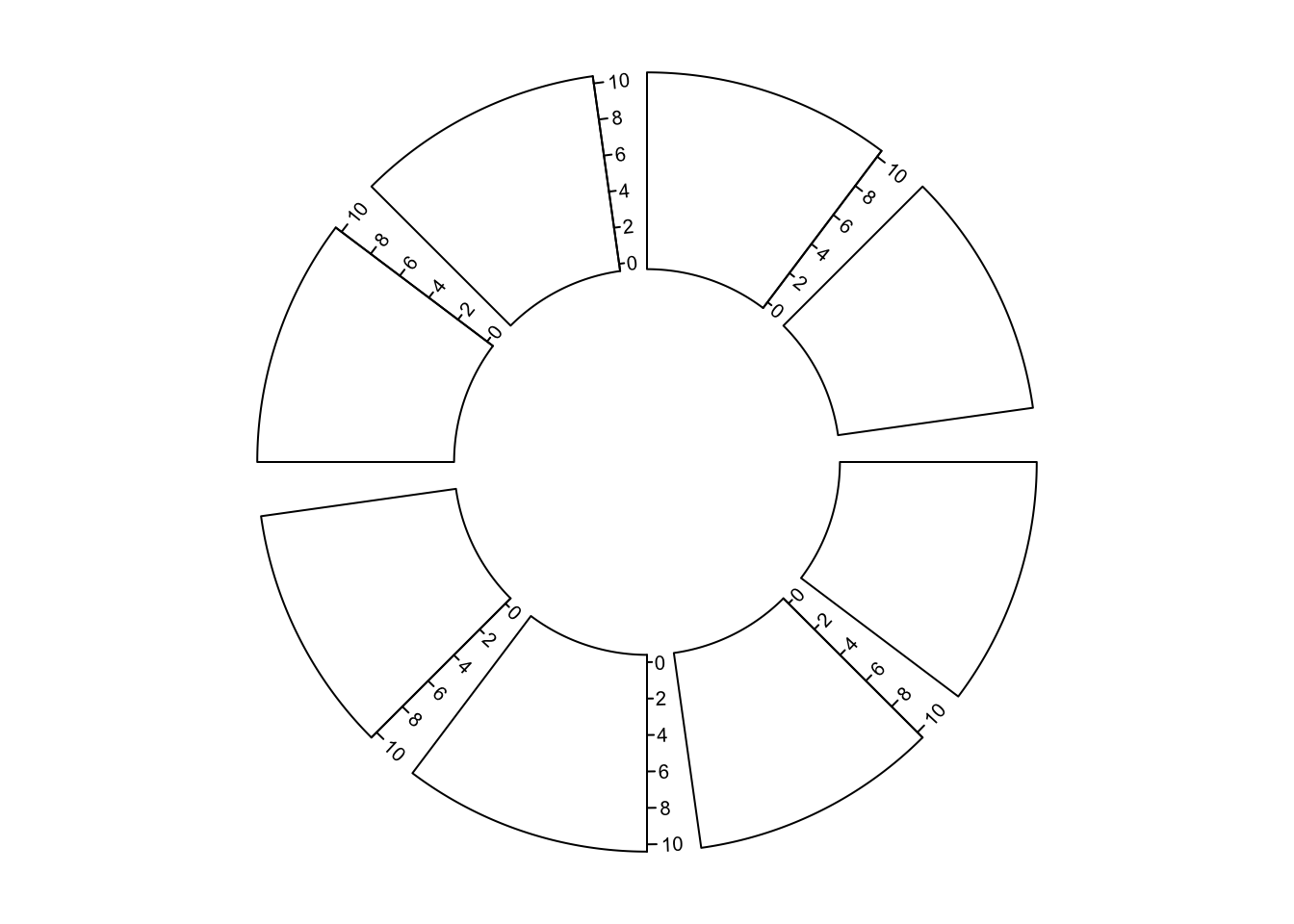Figure 3.8: Y-axes

## 3.8 Barplots, boxplots and violin plots

circos.barplot(), circos.boxplot() and circos.violin() are introduced together because the values on x-axes are the integer indices of bars, boxes or violins for which xlim should be properly set in circos.initialize().

For circular barplots, users can either specify a vector which generates a “normal” barplot, or a matrix which generates a stacked barplot (Figure 3.9).

par(mfrow = c(1, 2))
circos.initialize(letters[1:4], xlim = c(0, 10))
circos.track(ylim = c(0, 1), panel.fun = function(x, y) {
value = runif(10)
circos.barplot(value, 1:10 - 0.5, col = 1:10)
})
circos.track(ylim = c(-1, 1), panel.fun = function(x, y) {
value = runif(10, min = -1, max = 1)
circos.barplot(value, 1:10 - 0.5, col = ifelse(value > 0, 2, 3))
})
circos.clear()

circos.initialize(letters[1:4], xlim = c(0, 10))
circos.track(ylim = c(0, 4), panel.fun = function(x, y) {
value = matrix(runif(10*4), ncol = 4)
circos.barplot(value, 1:10 - 0.5, col = 2:5)
})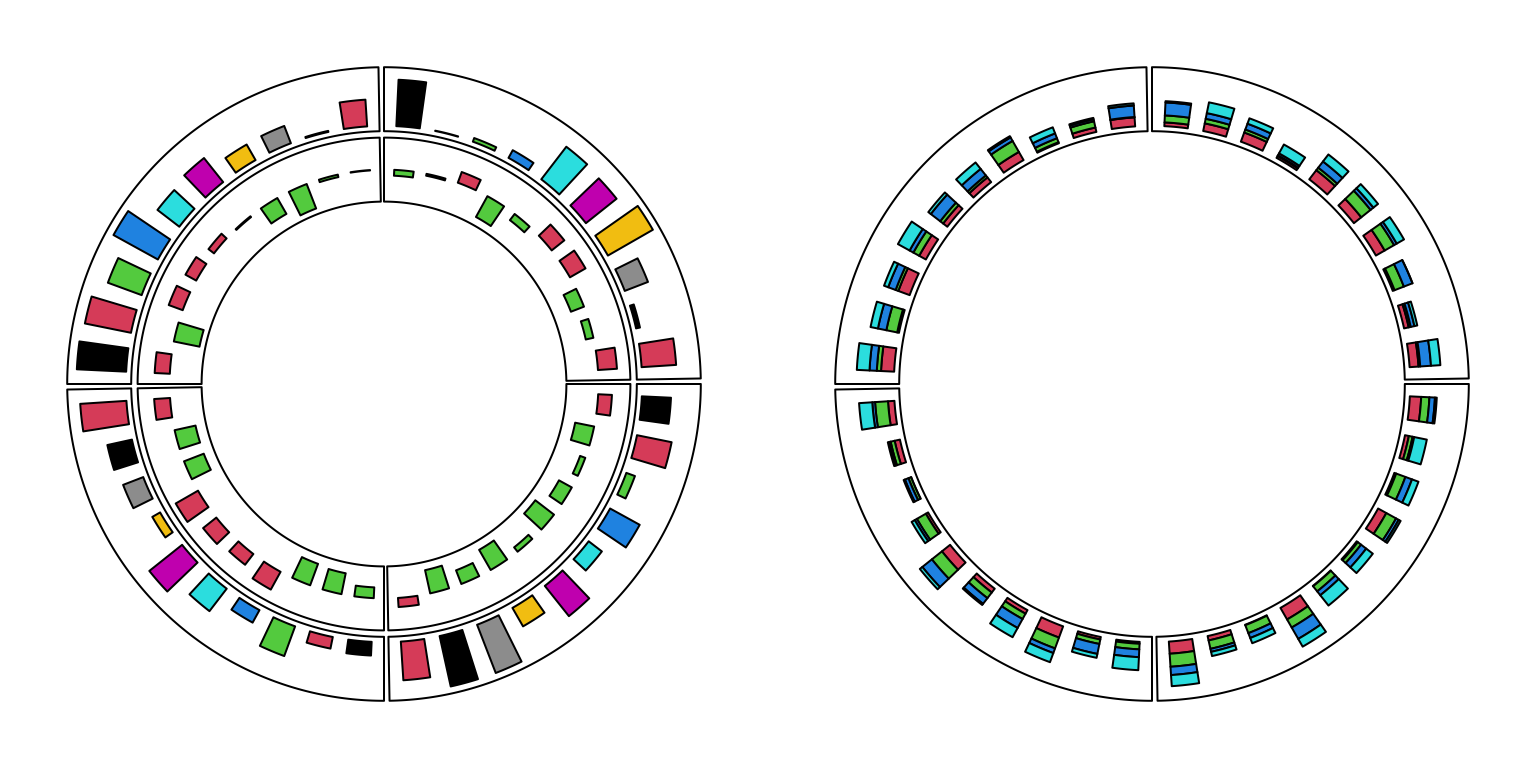Figure 3.9: Circular barplots.

circos.clear()

For circular boxplots, the boxes can be drawn one-by-one by providing a vector for each box, or drawn in batch with a list/matrix as input (Figure 3.10).

par(mfrow = c(1, 2))
circos.initialize(letters[1:4], xlim = c(0, 10))
circos.track(ylim = c(0, 1), panel.fun = function(x, y) {
for(pos in seq(0.5, 9.5, by = 1)) {
value = runif(10)
circos.boxplot(value, pos)
}
})
circos.clear()

circos.initialize(letters[1:4], xlim = c(0, 10))
circos.track(ylim = c(0, 1), panel.fun = function(x, y) {
value = replicate(runif(10), n = 10, simplify = FALSE)
circos.boxplot(value, 1:10 - 0.5, col = 1:10)
})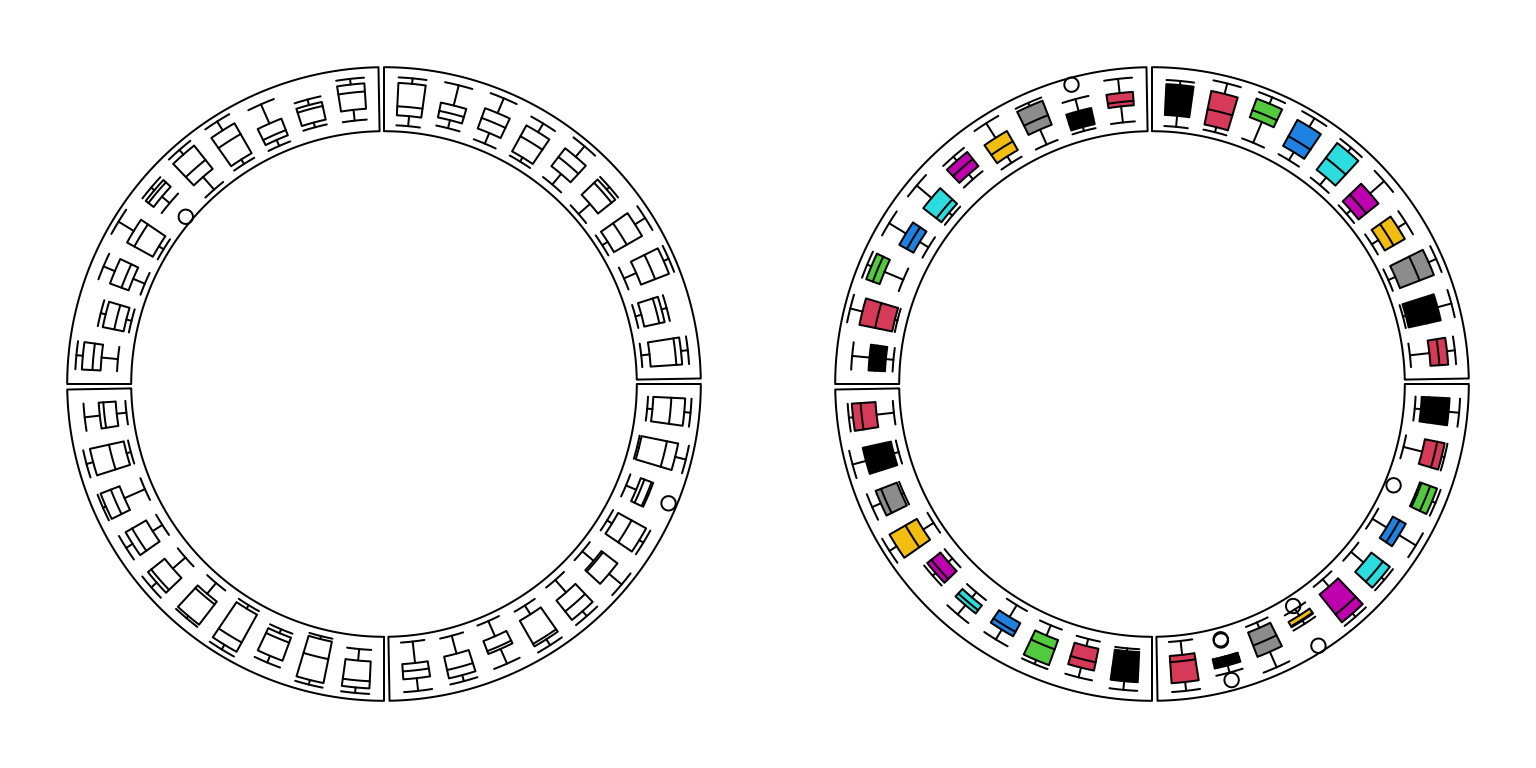Figure 3.10: Circular boxplots.

circos.clear()

For circular violin plots, the violins can be drawn one-by-one by providing a vector for each violin, or drawn in batch with a list/matrix as input (Figure 3.11).

Please note, to make it comparable between violins, max_density argument should be set.

par(mfrow = c(1, 2))
circos.initialize(letters[1:4], xlim = c(0, 10))
circos.track(ylim = c(0, 1), panel.fun = function(x, y) {
for(pos in seq(0.5, 9.5, by = 1)) {
value = runif(10)
circos.violin(value, pos)
}
})
circos.clear()

circos.initialize(letters[1:4], xlim = c(0, 10))
circos.track(ylim = c(0, 1), panel.fun = function(x, y) {
value = replicate(runif(10), n = 10, simplify = FALSE)
circos.violin(value, 1:10 - 0.5, col = 1:10)
})
## Warning in x/max_d * (violin_width/2): longer object length is not a multiple of
## shorter object length

## Warning in x/max_d * (violin_width/2): longer object length is not a multiple of
## shorter object length

## Warning in x/max_d * (violin_width/2): longer object length is not a multiple of
## shorter object length

## Warning in x/max_d * (violin_width/2): longer object length is not a multiple of
## shorter object length

## Warning in x/max_d * (violin_width/2): longer object length is not a multiple of
## shorter object length

## Warning in x/max_d * (violin_width/2): longer object length is not a multiple of
## shorter object length

## Warning in x/max_d * (violin_width/2): longer object length is not a multiple of
## shorter object length

## Warning in x/max_d * (violin_width/2): longer object length is not a multiple of
## shorter object length

## Warning in x/max_d * (violin_width/2): longer object length is not a multiple of
## shorter object length

## Warning in x/max_d * (violin_width/2): longer object length is not a multiple of
## shorter object length

## Warning in x/max_d * (violin_width/2): longer object length is not a multiple of
## shorter object length

## Warning in x/max_d * (violin_width/2): longer object length is not a multiple of
## shorter object length

## Warning in x/max_d * (violin_width/2): longer object length is not a multiple of
## shorter object length

## Warning in x/max_d * (violin_width/2): longer object length is not a multiple of
## shorter object length

## Warning in x/max_d * (violin_width/2): longer object length is not a multiple of
## shorter object length

## Warning in x/max_d * (violin_width/2): longer object length is not a multiple of
## shorter object length

## Warning in x/max_d * (violin_width/2): longer object length is not a multiple of
## shorter object length

## Warning in x/max_d * (violin_width/2): longer object length is not a multiple of
## shorter object length

## Warning in x/max_d * (violin_width/2): longer object length is not a multiple of
## shorter object length

## Warning in x/max_d * (violin_width/2): longer object length is not a multiple of
## shorter object length

## Warning in x/max_d * (violin_width/2): longer object length is not a multiple of
## shorter object length

## Warning in x/max_d * (violin_width/2): longer object length is not a multiple of
## shorter object length

## Warning in x/max_d * (violin_width/2): longer object length is not a multiple of
## shorter object length

## Warning in x/max_d * (violin_width/2): longer object length is not a multiple of
## shorter object length

## Warning in x/max_d * (violin_width/2): longer object length is not a multiple of
## shorter object length

## Warning in x/max_d * (violin_width/2): longer object length is not a multiple of
## shorter object length

## Warning in x/max_d * (violin_width/2): longer object length is not a multiple of
## shorter object length

## Warning in x/max_d * (violin_width/2): longer object length is not a multiple of
## shorter object length

## Warning in x/max_d * (violin_width/2): longer object length is not a multiple of
## shorter object length

## Warning in x/max_d * (violin_width/2): longer object length is not a multiple of
## shorter object length

## Warning in x/max_d * (violin_width/2): longer object length is not a multiple of
## shorter object length

## Warning in x/max_d * (violin_width/2): longer object length is not a multiple of
## shorter object length

## Warning in x/max_d * (violin_width/2): longer object length is not a multiple of
## shorter object length

## Warning in x/max_d * (violin_width/2): longer object length is not a multiple of
## shorter object length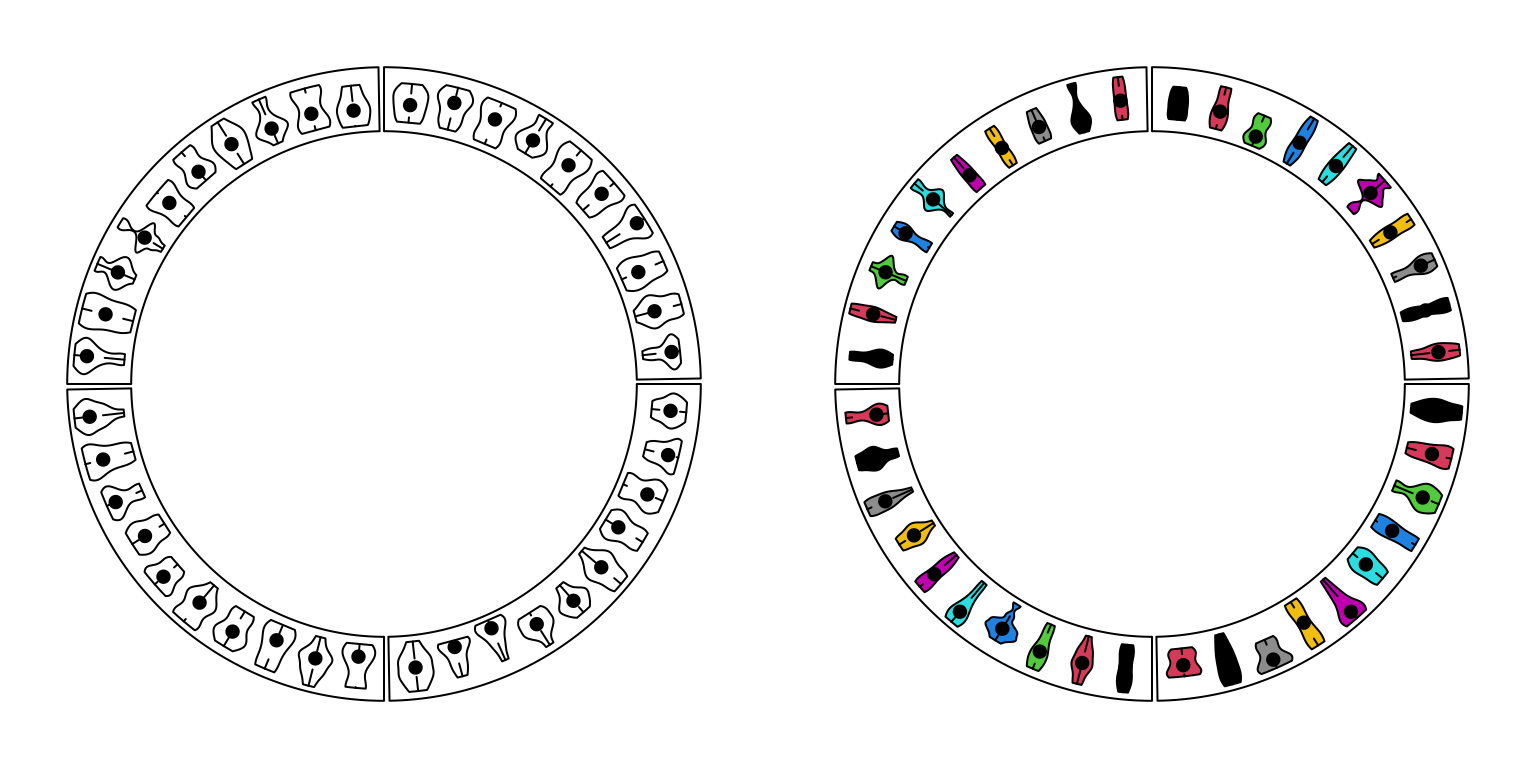Figure 3.11: Circular violin plots.

circos.clear()

## 3.9 Circular arrows

circos.arrow() draws circular arrows parallel to the circle. Since the arrow is always parallel to the circle, on x-direction, the start and end position of the arrow need to be defined while on the y-direction, only the position of the center of arrow needs to be defined. Also width controls the width of the arrow and the length is defined by x2 - x1. arrow.head.width and arrow.head.length control the size of the arrow head, and values are measured in the data coordinate in corresponding cell. tail controls the shape of the arrow tail. Note for width, arrow.head.width and arrow.head.length, the value can be set by e.g. mm_x(), mm_y() with absolute units. If users want to draw the arrows in the reversed direction, set arrow.position argument to start. See Figure 3.12.

par(mfrow = c(1, 2))
circos.initialize(letters[1:4], xlim = c(0, 1))
col = rand_color(4)
tail = c("point", "normal", "point", "normal")
circos.track(ylim = c(0, 1), panel.fun = function(x, y) {
circos.arrow(x1 = 0, x2 = 1, y = 0.5, width = 0.4,
col = col[CELL_META$sector.numeric.index], tail = tail[CELL_META$sector.numeric.index])
}, bg.border = NA, track.height = 0.4)
circos.clear()

circos.initialize(letters[1:4], xlim = c(0, 1))
tail = c("point", "normal", "point", "normal")
circos.track(ylim = c(0, 1), panel.fun = function(x, y) {
circos.arrow(x1 = 0, x2 = 1, y = 0.5, width = 0.4,
col = col[CELL_META$sector.numeric.index], tail = tail[CELL_META$sector.numeric.index],
arrow.position = "start")
}, bg.border = NA, track.height = 0.4)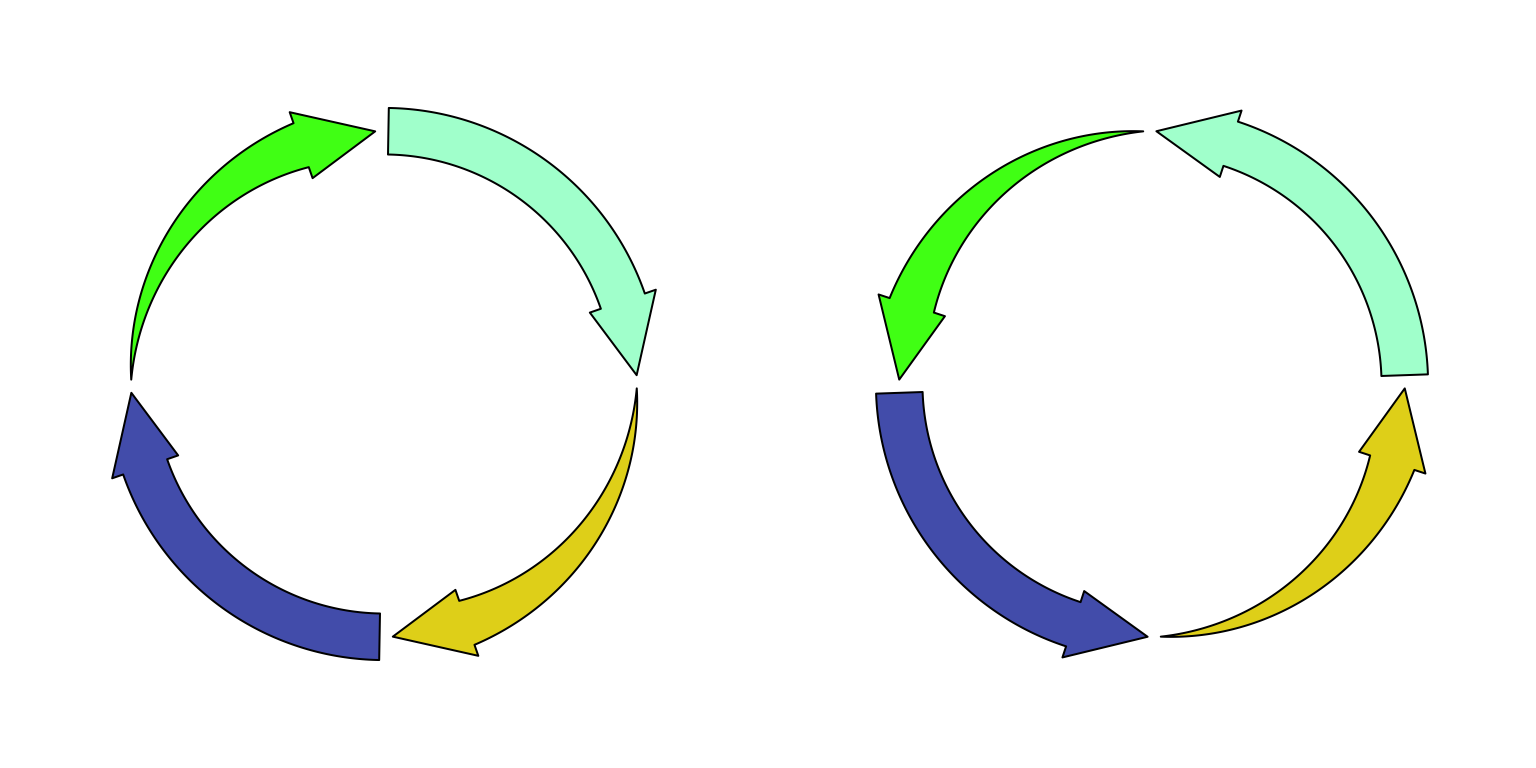Figure 3.12: Circular arrows.

circos.clear()

Circular arrows are useful to visualize events which happen in circular style, such as different phases in cell cycle. Following example code visualizes four phases in cell cycle where the width of sectors correspond to the hours in each phase (Figure 3.13). Also circular arrows can be used to visualize genes in circular genome where the arrows represent the orientation of the gene, such as mitochondrial genome or plasmid genome. Just remember if the gene is in the reverse strand or the negative strand, set arrow.position = "start" to draw the arrow in the other direction.

cell_cycle = data.frame(phase = factor(c("G1", "S", "G2", "M"), levels = c("G1", "S", "G2", "M")),
hour = c(11, 8, 4, 1))
color = c("#66C2A5", "#FC8D62", "#8DA0CB", "#E78AC3")
circos.par(start.degree = 90)
circos.initialize(cell_cycle$phase, xlim = cbind(rep(0, 4), cell_cycle$hour))
circos.track(ylim = c(0, 1), panel.fun = function(x, y) {
circos.arrow(CELL_META$xlim, CELL_META$xlim,
arrow.head.width = CELL_META$yrange*0.8, arrow.head.length = cm_x(0.5), col = color[CELL_META$sector.numeric.index])
circos.text(CELL_META$xcenter, CELL_META$ycenter, CELL_META$sector.index, facing = "downward") circos.axis(h = 1, major.at = seq(0, round(CELL_META$xlim)), minor.ticks = 1,
labels.cex = 0.6)
}, bg.border = NA, track.height = 0.3)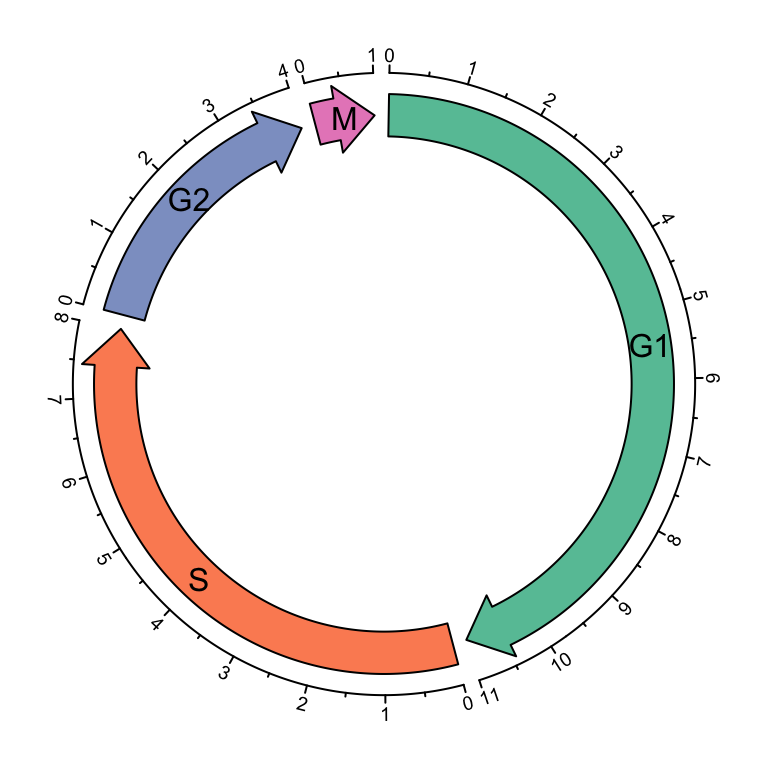Figure 3.13: Cell cycle.

circos.clear()

## 3.10 Labels

circos.labels() can be used to add a track of labels. This function creates two tracks, one for the connection lines and one for the labels. If two labels are too close and overlap, this function automatically adjusts the positions of neighouring labels. Check the following example (Figure 3.14).

par(mfrow = c(1, 2))
circos.initialize(sectors = letters[1:8], xlim = c(0, 1))
circos.track(ylim = c(0, 1))
circos.labels(c("a", "a", "b", "b"), x = c(0.1, 0.12, 0.4, 0.6), labels = c(0.1, 0.12, 0.4, 0.6))
circos.clear()

circos.initialize(sectors = letters[1:8], xlim = c(0, 1))
circos.labels(c("a", "a", "b", "b"), x = c(0.1, 0.12, 0.4, 0.6), labels = c(0.1, 0.12, 0.4, 0.6),
side = "outside")
circos.track(ylim = c(0, 1))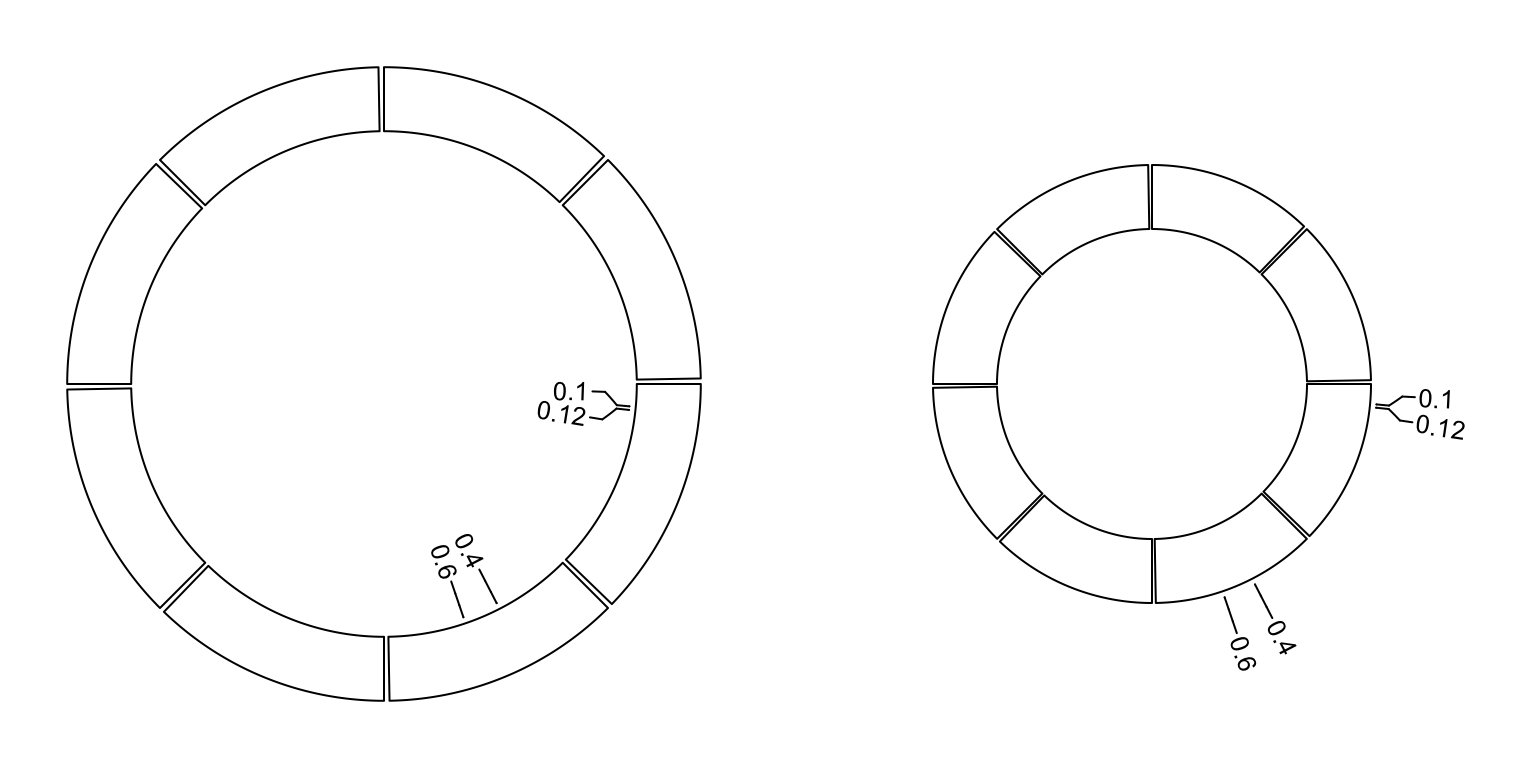Figure 3.14: Label track.

circos.clear()

## 3.11 Raster image

circos.raster() is used to add a raster image at a certain position in the circle with proper rotation. The first input variable should be a raster object or an object that can be converted by as.raster(). Facing of the image is controlled by facing and niceFacing arguments which are similar as in circos.text(). When value of facing is one of inside, outside, reverse.clockwise, clockwise and downward, the size of raster image should have absolute values which should be specified in the form of number- unit such as "20mm", "1.2cm" or "0.5inche". If only one of width and height is specified, the other one is automatically calculated by using the aspect ratio of the original image. Following example shows five types of facings of the raster image (figure 3.15).

library(png)
image = system.file("extdata", "Rlogo.png", package = "circlize")
circos.par(start.degree = 90)
circos.initialize(letters[1:5], xlim = c(0, 1))
all_facing_options = c("inside", "outside", "reverse.clockwise", "clockwise", "downward")
circos.track(ylim = c(0, 1), panel.fun = function(x, y) {
circos.raster(image, CELL_META$xcenter, CELL_META$ycenter, width = "1cm",
facing = all_facing_options[CELL_META$sector.numeric.index]) circos.text(CELL_META$xcenter, CELL_META$ycenter, all_facing_options[CELL_META$sector.numeric.index],
facing = "inside", niceFacing = TRUE)
})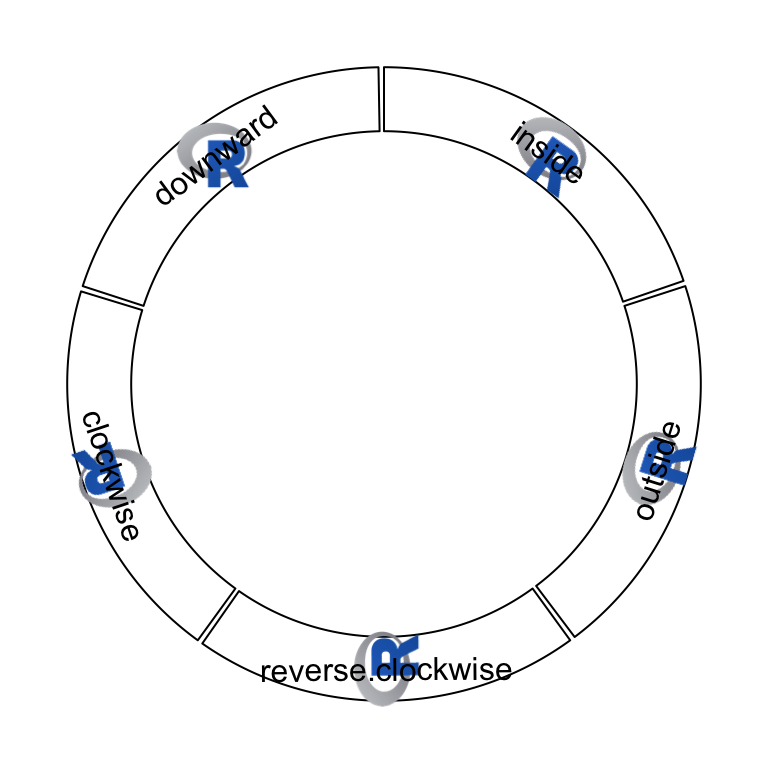Figure 3.15: Five facings of raster image.

circos.clear()

Also facing can be set to bending.inside and bending.outside that the image is filled to a circular rectangle. The strategy is to plot each original pixel as a small circular rectangle by circos.rect(), thus, the plotting is quite slow. If the original image is too huge, scaling argument can be set to reduce the size of the original image.

Following code draws the image of the cover of this book which is a circular style of Keith Haring’s doodle (Figure 3.16). The original source of the plot is from http://www.thegreenhead.com/imgs/keith-haring-double-retrospect-worlds-largest-jigsaw-puzzle-2.jpg.

load(system.file("extdata", "doodle.RData", package = "circlize"))
circos.par("cell.padding" = c(0, 0, 0, 0))
circos.initialize(letters[1:16], xlim = c(0, 1))
circos.track(ylim = c(0, 1), panel.fun = function(x, y) {
img = img_list[[CELL_META$sector.numeric.index]] circos.raster(img, CELL_META$xcenter, CELL_META$ycenter, width = CELL_META$xrange, height = CELL_META$yrange, facing = "bending.inside") }, track.height = 0.25, bg.border = NA) circos.track(ylim = c(0, 1), panel.fun = function(x, y) { img = img_list[[CELL_META$sector.numeric.index + 16]]
circos.raster(img, CELL_META$xcenter, CELL_META$ycenter,
width = CELL_META$xrange, height = CELL_META$yrange,
facing = "bending.inside")
}, track.height = 0.25, bg.border = NA)
circos.clear()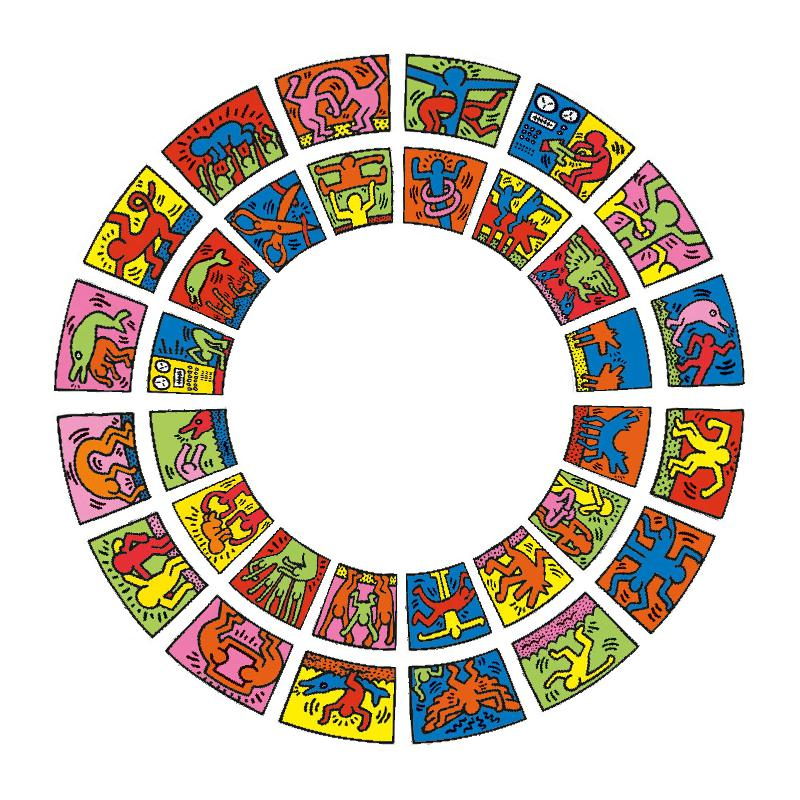Figure 3.16: Fill raster image to the cell.

## 3.13 Highlight sectors and tracks

draw.sector() draws sectors, rings or their parts. This function is useful if you want to highlight some parts of your circular plot. it needs arguments of the position of circle center (by default c(0, 0)), the start degree and the end degree for sectors, and radius for two edges (or one edge) which are up or bottom borders. draw.sector() is independent from the circular plot.

Possible usage of draw.sector() is as follows.

draw.sector(start.degree, end.degree, rou1)
draw.sector(start.degree, end.degree, rou1, rou2, center)
draw.sector(start.degree, end.degree, rou1, rou2, center, col, border, lwd, lty)

Directions from start.degree and end.degree is important for drawing sectors. By default, it is clock wise.

draw.sector(start.degree, end.degree, clock.wise = FALSE)

Following code shows examples of draw.sector() (Figure 3.24).

par(mar = c(1, 1, 1, 1))
plot(c(-1, 1), c(-1, 1), type = "n", axes = FALSE, ann = FALSE, asp = 1)
draw.sector(20, 0)
draw.sector(30, 60, rou1 = 0.8, rou2 = 0.5, clock.wise = FALSE, col = "#FF000080")
draw.sector(350, 1000, col = "#00FF0080", border = NA)
draw.sector(0, 180, rou1 = 0.25, center = c(-0.5, 0.5), border = 2, lwd = 2, lty = 2)
draw.sector(0, 360, rou1 = 0.7, rou2 = 0.6, col = "#0000FF80")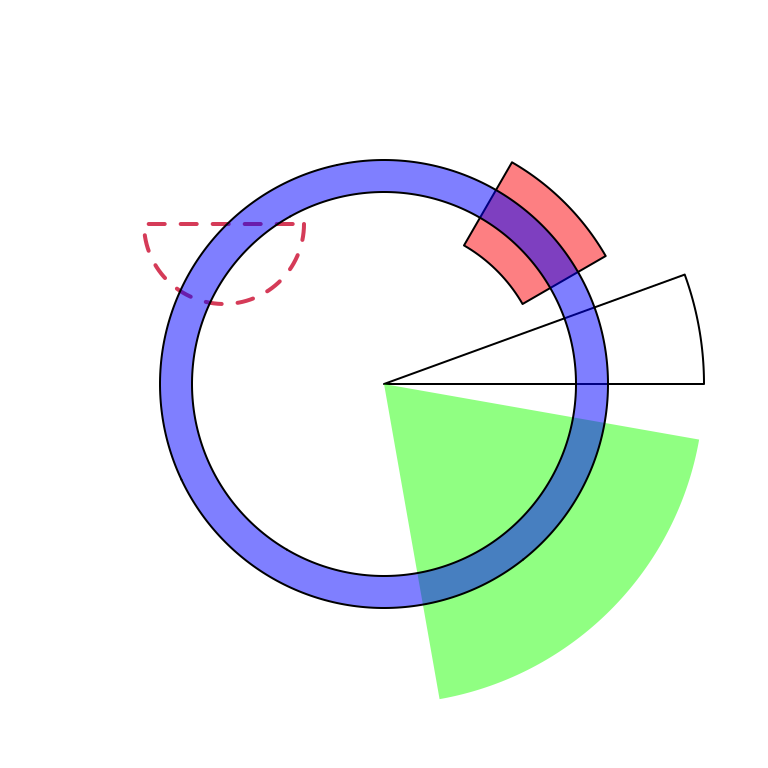Figure 3.24: General usage of ‘draw.sector().’

In order to highlight cells in the circular plot, we can use get.cell.meta.data() to get the information of positions of cells. E.g. the start degree and end degree can be obtained through cell.start.degree and cell.end.degree, and the position of the top border and bottom border can be obtained through cell.top.radius and cell.bottom.radius. Following code shows several examples to highlight sectors and tracks.

First we create a circular plot with eight sectors and three tracks.

sectors = letters[1:8]
circos.initialize(sectors, xlim = c(0, 1))
for(i in 1:3) {
circos.track(ylim = c(0, 1))
}
circos.info(plot = TRUE)

If we want to highlight sector a (Figure 3.25):

draw.sector(get.cell.meta.data("cell.start.degree", sector.index = "a"),
get.cell.meta.data("cell.end.degree", sector.index = "a"),
rou1 = get.cell.meta.data("cell.top.radius", track.index = 1),
col = "#FF000040")

If we want to highlight track 1 (Figure 3.25):

draw.sector(0, 360,
rou1 = get.cell.meta.data("cell.top.radius", track.index = 1),
rou2 = get.cell.meta.data("cell.bottom.radius", track.index = 1),
col = "#00FF0040")           

If we want to highlight track 2 and 3 in sector e and f (Figure 3.25):

draw.sector(get.cell.meta.data("cell.start.degree", sector.index = "e"),
get.cell.meta.data("cell.end.degree", sector.index = "f"),
rou1 = get.cell.meta.data("cell.top.radius", track.index = 2),
rou2 = get.cell.meta.data("cell.bottom.radius", track.index = 3),
col = "#0000FF40")

If we want to highlight specific regions such as a small region inside cell h:2, we can use circlize() to calculate the positions in the polar coordinate. But always keep in mind that x-axis in the cell are always clock wise. See Figure 3.25.

pos = circlize(c(0.2, 0.8), c(0.2, 0.8), sector.index = "h", track.index = 2)
draw.sector(pos[1, "theta"], pos[2, "theta"], pos[1, "rou"], pos[2, "rou"],
clock.wise = TRUE, col = "#00FFFF40")
circos.clear()Figure 3.25: Highlight sectors and tracks.

If the purpose is to simply highlight complete cells, there is a helper function highlight.sector() for which you only need to specify index for sectors and tracks that you want to to highlight. Paddings of the highligted regions can be set by padding argument which should contain four values representing ratios of the width or height of the highlighted region (Figure 3.26).

One advantage of highlight.sector() is that it supports to add text in the highlighted regions. By default, the text is drawn at that center of the highlighted region. The position on the radical direction can be set by text.vjust argument either by a numeric value or a string in form of "2 inches" or "-1.2cm".

sectors = letters[1:8]
circos.initialize(sectors, xlim = c(0, 1))
for(i in 1:4) {
circos.track(ylim = c(0, 1))
}
circos.info(plot = TRUE)

highlight.sector(c("a", "h"), track.index = 1, text = "a and h belong to a same group",
facing = "bending.inside", niceFacing = TRUE, text.vjust = "6mm", cex = 0.8)
highlight.sector("c", col = "#00FF0040")
highlight.sector("d", col = NA, border = "red", lwd = 2)
highlight.sector("e", col = "#0000FF40", track.index = c(2, 3))
highlight.sector(c("f", "g"), col = NA, border = "green",
lwd = 2, track.index = c(2, 3), padding = c(0.1, 0.1, 0.1, 0.1))
highlight.sector(sectors, col = "#FFFF0040", track.index = 4)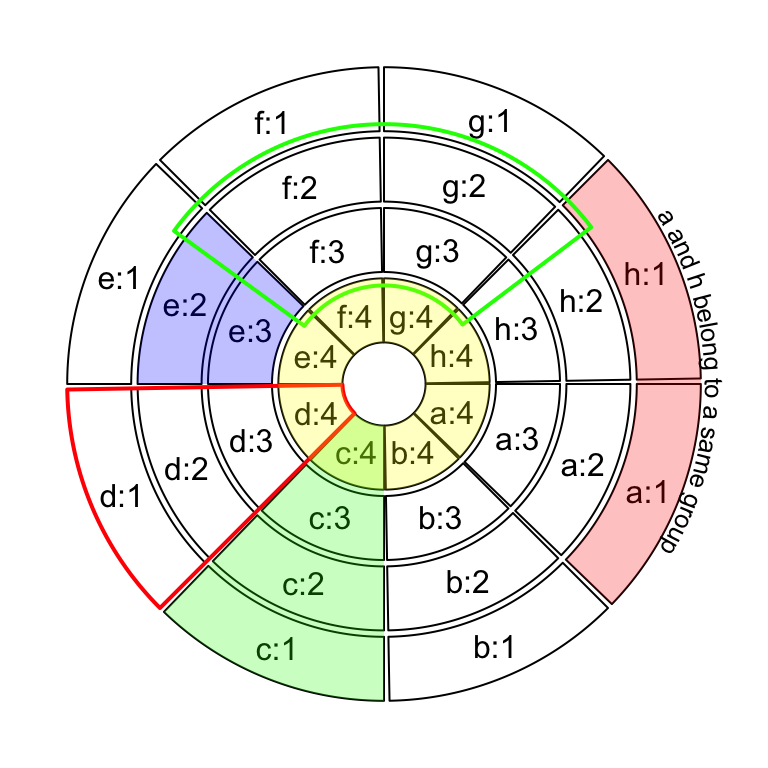Figure 3.26: Highlight sectors.

circos.clear()

## 3.14 Work together with the base graphic system

circlize is built on the base R graphic system, then, of course the base graphic functions can be used in combination with circlize functions. On the other hand, circlize() converts data points from the data coordinates to the canvas coordinates where the base graphic function can be directly applied.

Normally, the base functions such as title(), text(), legend() can be used to add extra information on the plot (Figure 3.27).

Sometimes, when the text or other graphics are far from the circle, you may set par(xpd = NA) so that the plotting is not clipped.

sectors = letters[1:4]
circos.initialize(sectors, xlim = c(0, 1))
circos.track(ylim = c(0, 1), panel.fun = function(x, y) {
circos.points(1:20/20, 1:20/20)
})
text(0, 0, "This is\nthe center", cex = 1.5)
legend("bottomleft", pch = 1, legend = "This is the legend")
title("This is the title")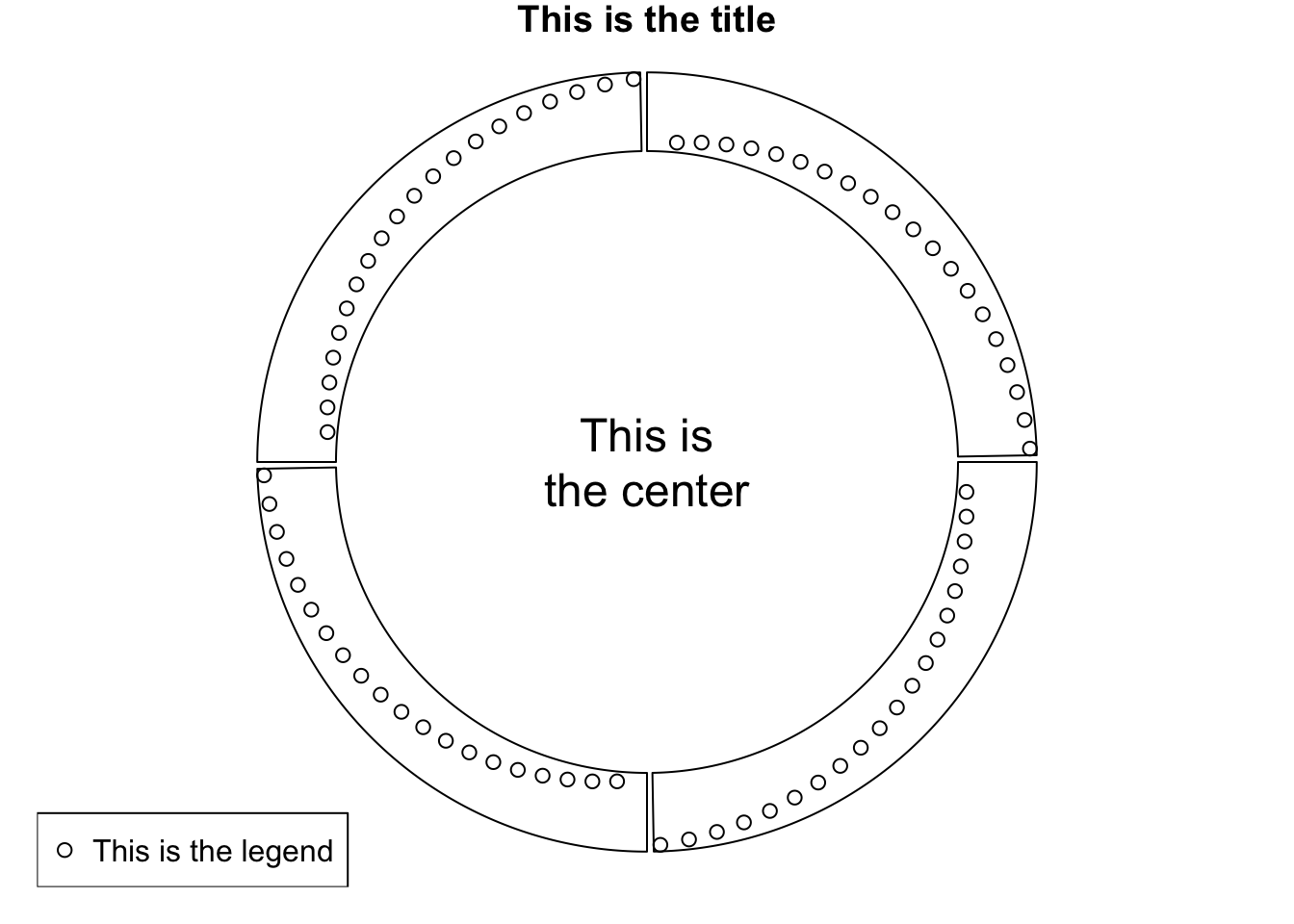Figure 3.27: Work with base graphic functions.

circos.clear()